##### PostgreSQL UPPER() Function With Practical Examples

PostgreSQL offers multiple built-in functions to perform different functionalities on the strings. One of the most commonly used functions/operations when working with strings is case conversion. Sometimes users must convert the given strings from lowercase to uppercase or vice versa to fulfill some specific purpose. To deal with such cases, Postgres offers some built-in functions such as LOWER(), UPPER(), and INITCAP(). Postgres' UPPER() function accepts a string as an argument and converts the string’s case to upper.

This blog post demonstrates the working of the UPPER() function in Postgres via practical examples. So, let’s begin.

How to Use the UPPER() Function in Postgres?

In PostgreSQL, letter case conversion from lower to upper or upper to lower is a very common task while working with strings. A string can be converted to uppercase in Postgres using the UPPER() function.

Syntax

To use the UPPER() function in Postgres, users must follow the below syntax:

`UPPER(input_string);`

Here, input_string represents a string to be converted into uppercase. The data type of the input_string can be CHAR, VARCHAR, or TEXT.

Let’s comprehend the working of the UPPER() function through practical examples.

Example 1: How to Convert a String Into Upper Case Letters?

Let’s pass the string “welcome to commandprompt.com” to the UPPER() function and see how it works:

`SELECT UPPER('welcome to commandprompt.com');`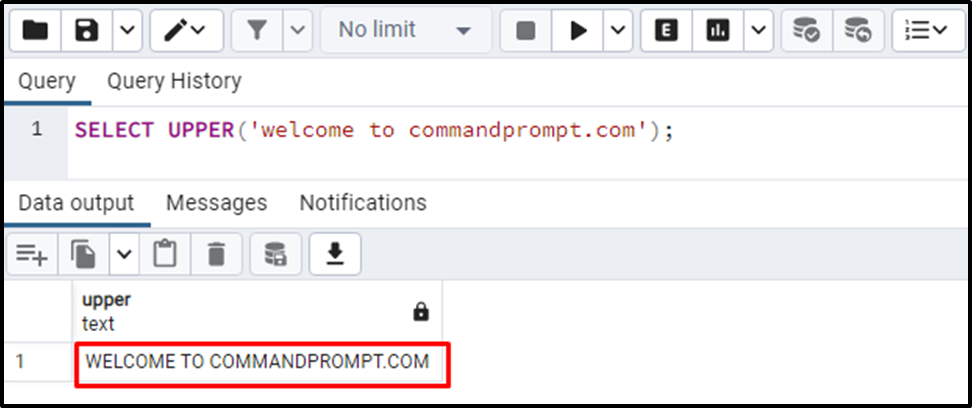The output shows that the given string has been converted into uppercase successfully.

Example 2: How to Use the UPPER() Function on Table’s Data?

Firstly, we will create a sample table named student_bio with three columns: student_id, student_name, and student_email:

```CREATE TABLE student_bio(
student_id INT,
student_name TEXT,
student_email VARCHAR
);```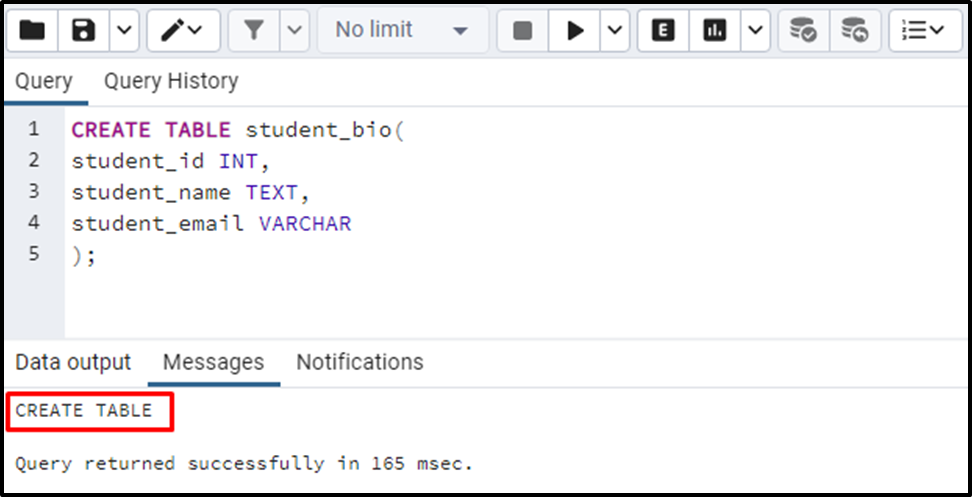A table named “student_bio” with the three columns has been created successfully. To insert data into the “student_bio” table, we will utilize the following command:

```INSERT INTO student_bio(student_id, student_name, student_email)
VALUES (1, 'anna', 'anna123@xyz.com'),
(2, 'stephen', 'stephen321@abc.com'),
(3, 'henry', 'henry098@abc.com'),
(4, 'natie', 'natie@xyz.com'),
(5, 'mike', 'mike@xyz.com');```

Let’s utilize the UPPER() function to convert the student_name column into uppercase. To do so, we will utilize the UPPER() function as follows:

```SELECT student_name, UPPER(student_name)
FROM student_bio;```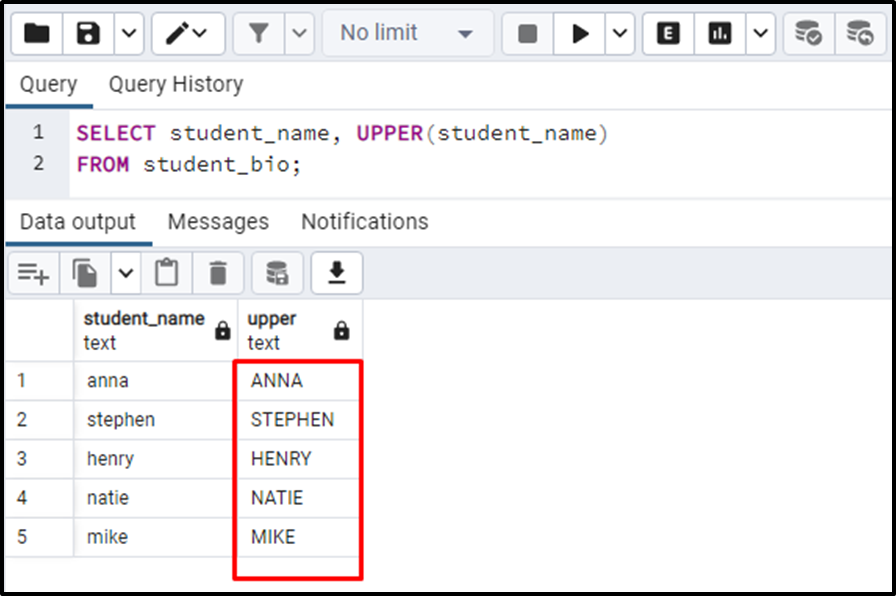The output authenticates that the “student_name” column has been converted into uppercase letters successfully.

Example 3: How to Use UPPER() Function With WHERE Clause in Postgres?

You can use the UPPER() function with the WHERE clause to convert some specific strings into uppercase:

```SELECT student_name, UPPER(student_name)
FROM student_bio
WHERE student_id >= 3;```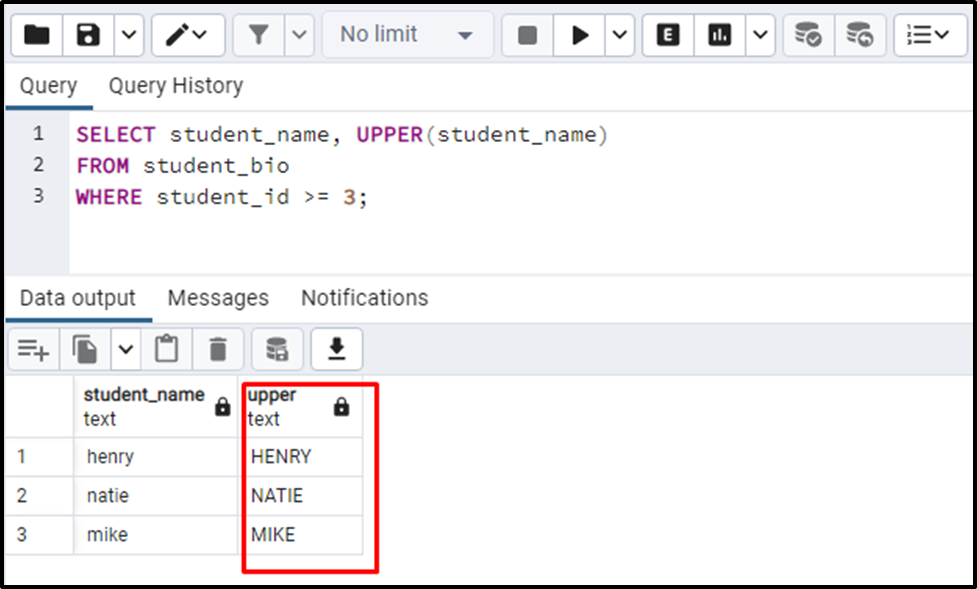The output clarifies that the UPPER() function converts all those student names into uppercase that satisfies the given criteria.

Example 4: Pass Integer Value to UPPER() Function in Postgres

Let’s utilize the UPPER() function on the student_id column to see how it deals with the integer values:

```SELECT UPPER(student_id)
FROM student_bio;```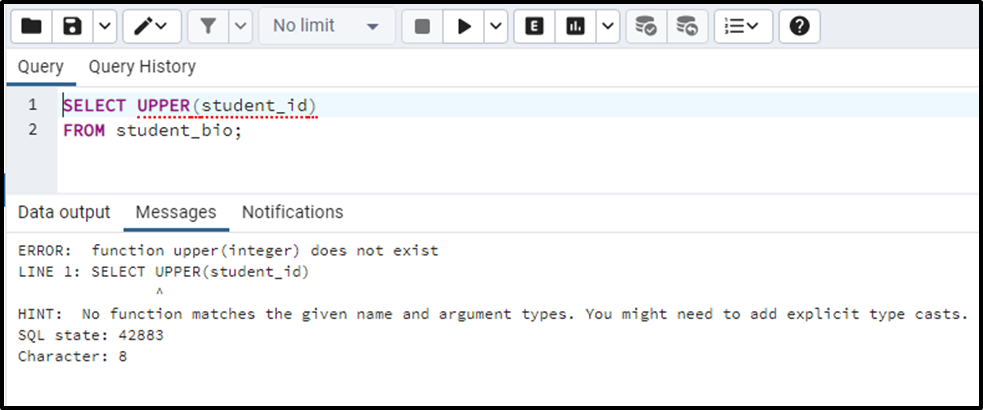When we passed an integer to the UPPER() function, Postgres threw an error.

That’s all from this guide!

Conclusion

Postgres' UPPER() function accepts a string as an argument and converts the string’s case to upper. It can accept any character string, such as CHAR, TEXT, and VARCHAR. Passing anything other than string will throw an error. This blog post has demonstrated various use cases of the Postgres UPPER() function through practical examples.